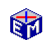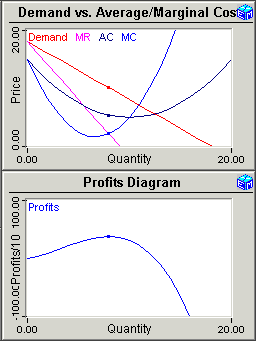Monopoly / Monopolistic Competitiion

The Monopoly application has the same average cost curve and marginal cost curves as the Perfect Competition application. The introductory steps explain that the difference lies with the downward-sloping demand curve and the downward-sloping marginal revenue curve.Model Link:   Monopolistic Competition
Printable PDF ExercisesFollowing the example of Perfect Competition, the first step is to find the profit-maximizing position.  You adjust the quantity produced manually to see first-hand how a firm facing a downward-sloping demand curve maximizes profits by producing where the marginal cost equals the marginal revenue.  You then use a built-in iterative procedure to automatically maximize profits.

To illustrate the behavior of the firm in a changing environment, you study how the profit maximizing quantity changes when changes in technology shift the average cost and marginal cost curves.

Classic Economic Models

Microeconomics

Introduction
Overview of Micro Models

Macroeconomics

Introduction
Overview of Macro Models

Resources